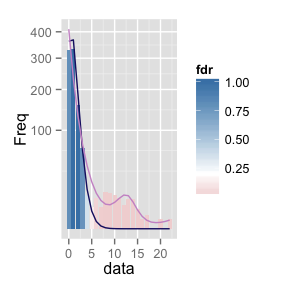In this document, we (1) introduce an extension to the Local False Discovery Rate (Local FDR) methodology and (2) explain how it is a natural approach to mutation data.

This method was designed in the context of count data, where there are two regimes of interest – the prevailing one with small counts, and a rarer one with much higher valued counts. Our specific motivation comes from the detection of APOBEC mutations, which are important in understanding the evolution fo HIV. To determine the number of mutations to use as a cutoff for declaring a mutation an APOBEC mutation, we adopt a method originally developed in the multiple testing community, called the Local False Discovery Rate . This method is designed for the situation where, from a large collection of mostly uninteresting measurements, we need to identify a few that stand out’’ and are worthy of followup study. For example, most genes will have relatively few mutations, but a few that have an unusually large number are reasonable candidates for being APOBEC mutations.

Formally, we assume the data is drawn from a mixture of two distributions, $$f_{0}$$ and $$f_{1}$$, the null and alternative, respectively. The null is more common than the alternative, which is modeled by assuming that the mixture proportion $$\pi_{0}$$ for the null component is close to 1. So, the observed data density is

and the probability that a point comes from the null component given an observed value of $$x$$ is, by Bayes rule,

The main idea of he Local FDR algorithm is to estimate both $$\pi_{0}$$ and the ratio $$\frac{f_{0}\left(x\right)}{f\left(x\right)}$$. In the case that there are many null observations, this can be done reliably, through maximum likelihood or generalized linear models, see for example . An implementation of this method for the case used by this paper is provided in our R library, .

The Local FDR method is most commonly used to identify genes with large effects in gene expression experiments, but there is an analogy with the identification of APOBEC mutations.

In the gene expression application, most genes do not display any differential expression between treatment and control groups, while a small subset may exhibit some treatment effect. To measure treatment effects, it is common to use $$z$$-statistics, which will be standard normal in the null genes (this is $$f_{0}$$), and are assumed normal with unknown, nonzero means in those of interest (this is $$f_{1}$$). The proportion of genes that are null is $$\pi_{0}$$.

In our APOBEC mutation scenario, most genes are assumed to have a mutation count drawn from a Poisson distribution with low mean ($$f_{0}$$), while those resulting from APOBEC are drawn from a Poisson with larger mean ($$f_{1}$$). The small probability and many opportunities for mutation together make this approximation by a mixture of Poisson distributions reasonable. One slight difference between gene expression setup is that earlier we assumed that the null density was from a known density (a standard normal), while in the current scenario we only have an approximate idea of the null density (a Poisson with small mean). Fortunately, a variation of the Local FDR method enables estimation of an empirical null, see Section 6 of .

In this section, we provide the code for performing the Local FDR estimation described in the previous sections, using simulated data.

First, we load the required libraries.

library("LocFDRPois")
n0 <- 900
n1 <- 100
lambda0 <- 1
lambda1 <- 10
sim_data <- c(rpois(n0, lambda0), rpois(n1, lambda1))
To perform Local FDR estimation, the key function is in package . It returns
SummarizeLocfdr(sim_data)
## $pi0 ##  0.9051895 ## ##$lambda0
##  1.014957
##
## $locfdr_res ## data Freq f0 f fdr ## 1 0 328 3.624181e+02 410.6970533 7.987810e-01 ## 2 1 333 3.678387e+02 232.0498567 1.000000e+00 ## 3 2 158 1.866702e+02 130.8456446 1.000000e+00 ## 4 3 67 6.315407e+01 73.4807763 7.779776e-01 ## 5 4 12 1.602466e+01 41.0150218 3.536596e-01 ## 6 5 5 3.252869e+00 22.7660944 1.293354e-01 ## 7 6 10 5.502535e-01 13.0599628 3.813822e-02 ## 8 7 5 7.978337e-02 8.2859804 8.715815e-03 ## 9 8 14 1.012209e-02 6.2267593 1.471456e-03 ## 10 9 13 1.141498e-03 5.9337244 1.741354e-04 ## 11 10 12 1.158571e-04 7.1808695 1.460445e-05 ## 12 11 10 1.069000e-05 9.5226903 1.016149e-06 ## 13 12 6 9.041571e-07 11.7022950 6.993786e-08 ## 14 13 9 7.059080e-08 11.2695604 5.669968e-09 ## 15 14 9 5.117616e-09 7.4123337 6.249600e-10 ## 16 15 4 3.462773e-10 3.6262558 8.643807e-11 ## 17 16 1 2.196603e-11 1.5879145 1.252172e-11 ## 18 17 2 1.311446e-12 0.7493337 1.584216e-12 ## 19 18 0 7.394781e-14 0.4574734 1.463184e-13 ## 20 19 0 3.950202e-15 0.3878084 9.220226e-15 ## 21 20 1 2.004642e-16 0.4236006 4.283708e-16 ## 22 21 0 9.688693e-18 0.5478830 1.600726e-17 ## 23 22 1 4.469821e-19 0.7711077 5.247042e-19 ## ##$locfdr_fig